# Detect Anomalies Using Wavelet Scattering with Autoencoders

This example shows how to use the wavelet scattering transform with both LSTM and convolutional autoencoders to develop an alert system for predictive maintenance. The example compares wavelet scattering transform+autoencoder and raw data+autoencoder approaches.

### Data

The dataset is collected from a 2MW wind turbine high-speed shaft driven by a 20-tooth pinion gear . A vibration signal of 6 seconds was acquired at a sample rate of 97,656 Hz each day for 50 consecutive days from 2013-03-07 to 2013-04-25. There are two measurements on 2013-03-17, which are treated as two days in this example. Each timeseries consists of 585,936 samples. An inner race fault developed and caused the failure of the bearing across the 50-day recording period.

Obtain the data from https://github.com/mathworks/WindTurbineHighSpeedBearingPrognosis-Data. You can download the entire repository as a zip file and save it in a folder where you have write permission. The commands in this example assume that you have downloaded the data in the folder MATLAB designates as `tempdir`. If you choose to use a different folder, change the value of `parentDir` below. After downloading the zip file, unzip the data using this command.

```parentDir = tempdir; if exist(fullfile(parentDir,'WindTurbineHighSpeedBearingPrognosis-Data-main.zip'),'file') unzip(fullfile(parentDir,'WindTurbineHighSpeedBearingPrognosis-Data-main.zip'),parentDir) else error("File not found. "+ ... "\nManually download the repository as a .zip file from GitHub. "+ ... "Confirm the .zip file is in: \n%s",parentDir) end```

If you prefer to use Git commands to clone the repository, the folder does not include the "`-main`" designation and the data is placed in a `WindTurbineHighSpeedBearingPrognosis-Data` folder.

### Signal Datastore

Unzipping the `WindTurbineHighSpeedBearingPrognosis-Data-main.zip` file creates a folder with 50 `.mat` files. Each `.mat` file contains two variables: `tach` and `vibration`. This example utilizes only the vibration data. Accordingly, create a signal datastore to read only the vibration data from the `.mat` files.

```filepath = fullfile(parentDir,'WindTurbineHighSpeedBearingPrognosis-Data-main'); sds = signalDatastore(filepath,SignalVariableNames = "vibration");```

Because there are only 50 records in this dataset, read all the data into memory at once.

`allSignals = readall(sds);`

The data records are relatively long at 585,936 samples. As a first attempt at data reduction, examine the spectral characteristics of a random sample of 6 signals. Use the maximal overlap wavelet packet transform with the default wavelet and level. This results in an orthogonal decomposition of the signal's energy into passbands of width $Fs/{2}^{5}$ where Fs is the sample rate. Plot the relative energy of each signal as a function of the passband. Print the percentage of signal energy below 1/4 of the sampling frequency.

```rng default fs = 97656; rdIdx = randperm(length(allSignals),6); figure tiledlayout(2,3) for ii = 1:6 nexttile [~,~,f,~,re] = modwpt(allSignals{ii}); bar((f.*fs)/1e3,re.*100) sprintf('%2.2f',sum(re(f <= 1/4))*100) record = rdIdx(ii); title({'Passband Energy:' ; ['Record ' num2str(record)]}); xlabel("kHz") ylabel("Percentage Energy") end```
```ans = '96.73' ```
```ans = '95.84' ```
```ans = '96.00' ```
```ans = '95.61' ```
```ans = '95.77' ```
```ans = '95.95' ```In these samples, approximately 96% of the signal energy is below the cutoff frequency of 24.414 kHz required for downsampling the signal length by a factor of 2. Examination of all signals in the data set reveals that all records have between 94.7 and 98.2 percent of their energy below 24.414 kHz. Resample all signals at 1/2 the original sample rate and adjust the sample rate accordingly.

```allSignals = cellfun(@(x)resample(x,1,2),allSignals,UniformOutput = false); fs = fs/2;```

The data in this example are unlabeled. To obtain an idea of how the data evolves over the 50-day period, create a cumulative plot.

```tstart = 0; figure for ii = 1:length(allSignals) t = (1:length(allSignals{ii}))./fs + tstart; hold on plot(t,allSignals{ii}) tstart = t(end); end hold off title("Cumulative Wind-Turbine Vibration Monitoring") xlabel("Time (sec) -- 6 seconds per day for 50 days") ylabel("Voltage")```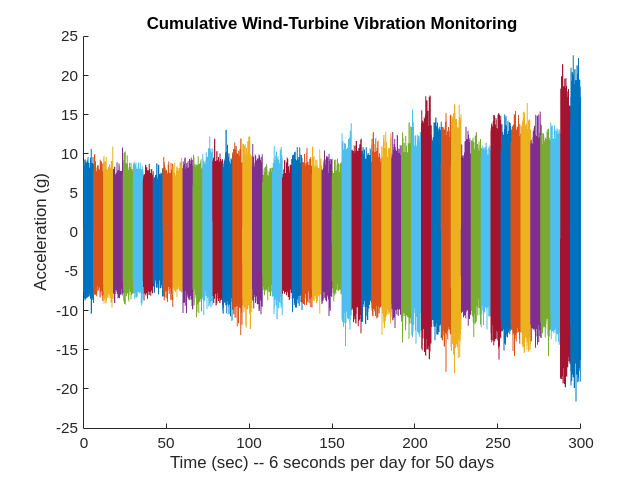From the preceding plot, it is evident that the amount of vibration in the data appears to be gradually increasing on average over time. This appears especially evident after 200 seconds of recording, which corresponds to the period beyond 33 days. While this average trend is evident, note that for individual recordings this is not always the case. Some recordings near the end of the 50-day period exhibit behavior similar to recordings nearer the beginning.

### Data Preparation and Feature Extraction

Split each 6 second recording into 11 separate recordings of 1 second each overlapped by 0.5 seconds. Cast the data to single precision.

```frameSize = 1*fs; frameRate = 0.5*fs; nframe = (length(allSignals{1})-frameSize)/frameRate + 1; nday = length(allSignals); myXAll = zeros(frameSize,nframe*nday); XAll = zeros(frameSize,nframe*nday); colIdx = 1; for ii = 1:length(allSignals) XAll(:,colIdx:colIdx+nframe-1) = buffer(allSignals{ii},frameSize,... frameRate,'nodelay'); colIdx = colIdx+nframe; end XAll = single(XAll);```

The resulting `XAll` has 550 columns representing 11 recordings for each of the 50 days. Each column of `XAll` has 48,828 samples.

Set up the wavelet scattering network. Set the invariance scale to 0.2 seconds and the number of wavelet filters per octave to be 4 in the first layer and 1 in the second layer. Set the oversampling factor to 1 and optimize the path, or channel computation of the network.

```N = size(XAll,1); sn = waveletScattering(SignalLength = N, SamplingFrequency = fs,... InvarianceScale = 0.2, QualityFactors = [4 1],... OptimizePath = true, OversamplingFactor = 1, Precision = 'single');```

Display the number of scattering coefficients per path (channel) and the total number of channels.

```[~,pathbyLev] = paths(sn); Ncfs = numCoefficients(sn)```
```Ncfs = 48 ```
`sum(pathbyLev)`
```ans = 180 ```

There are 180 channels in the output of the scattering transform. However, there are only 48 time samples. This means the amount of data for each signal is reduced by nearly a factor of 6.

Obtain all the wavelet scattering features for the data. In this example, we omit the zero-th order scattering coefficients. As a result, the number of channels reduces by 1 to 179. If you have a supported GPU, you can accelerate the scattering transform by setting `useGPU` to `true`. Otherwise, set `useGPU = false`.

```useGPU = true; if useGPU XAll = gpuArray(XAll); end SAll = sn.featureMatrix(XAll); SAll = SAll(2:end,:,:); npaths = size(SAll,1); scatfeatures = squeeze(num2cell(SAll,[1,2]));```

`scatfeatures` is a cell array with 550 elements. Each element contains the scattering transform of a one-second recording. Because this data is unlabeled, we are uncertain which records are indicative of normal operation and which records indicate the presence of the inner-race fault. All we know from the data description is that an inner-race fault develops sometime over the 50-day period.

Accordingly, we construct our training and validation sets from the earliest recordings to maximize the probability that these sets contain only recordings without the presence of the inner-race fault. The first 66 records (6 days) are used to form the training set and the next 44 recordings (4 days) are used to form the validation set. The remaining 440 recordings (40 days) are held out as the test set.

```ntrain = 6; trainscatFeatures = scatfeatures(1:ntrain*nframe); validationscatFeatures = scatfeatures(ntrain*nframe+1:ntrain*nframe+4*nframe); testscatFeatures = scatfeatures((ntrain*nframe+4*nframe+1):end);```

In this example, we compare the scattering transform with networks fit to the absolute values of the raw time series. The absolute values are used based on the observation that the amplitude of the vibrations appears to increase on average over the 50-day period.

```rawfeatures = num2cell(XAll,1)'; rawfeatures = cellfun(@transpose,rawfeatures,UniformOutput = false); rawABSfeatures = cellfun(@abs,rawfeatures,UniformOutput = false); ntrain = 6; trainrFeatures = rawABSfeatures(1:ntrain*nframe); validationrFeatures = rawABSfeatures(ntrain*nframe+1:ntrain*nframe+4*nframe); testrFeatures = rawABSfeatures((ntrain*nframe+4*nframe+1):end);```

### Deep Networks

In this example, two deep-learning autoencoders are used: an LSTM and a convolutional autoencoder.

The LSTM autoencoder uses Z-score normalization at the input followed by two LSTM layers at the encoding stage consisting of 179 channels and `floor(179/2)` channels respectively. The final LSTM layer in the encoder only outputs from the last timestep cell, `OutputMode = "last"`. Subsequently, a custom layer, `repeatVectorLayer`, is used to replicate this sample for the next LSTM layer.

```Ntimesteps = Ncfs; lstmAutoEncoder = [ sequenceInputLayer(npaths, Normalization = "zscore",... Name = "input", MinLength = Ntimesteps) lstmLayer(npaths, Name = "lstm1a") reluLayer(Name = "relu1") lstmLayer(floor(npaths/2), Name = "lstm2a", OutputMode = "last") dropoutLayer(0.2, Name = "drop1") reluLayer(Name = "relu2") repeatVectorLayer(Ntimesteps) lstmLayer(floor(npaths/2), Name = "lstm2b") dropoutLayer(0.2,Name = "drop2") reluLayer(Name = "relu3") lstmLayer(npaths, Name = "lstm1b") reluLayer(Name = "relu4") regressionLayer(Name = "regression") ];```

Train the autoencoder for 200 epochs. Use a minibatch size of 16, shuffle the training data every epoch. Output the network with the best validation loss.

```options = trainingOptions('adam', ... 'MaxEpochs',200, ... 'MiniBatchSize',16, ... 'Shuffle','every-epoch',... 'ValidationData',{validationscatFeatures,validationscatFeatures},... 'Plots','training-progress',... 'Verbose', false,... 'OutputNetwork','best-validation-loss'); scatLSTMAutoencoder = trainNetwork(trainscatFeatures,trainscatFeatures,... lstmAutoEncoder,options);```### Threshold determination

There is no definitive rule for determining the threshold to use in anomaly detection. In this example, the mean-absolute error (MAE) is used due to the robust behavior of the L1 norm with respect to outliers. First, compute the MAE errors for the training, validation, and test data.

```ypredTrain = cellfun(@(x)predict(scatLSTMAutoencoder,x),trainscatFeatures,'UniformOutput',false); maeTrain = cellfun(@(x,y)maeLoss(x,y),ypredTrain,trainscatFeatures); ypredValidation = cellfun(@(x)predict(scatLSTMAutoencoder,x),validationscatFeatures,'UniformOutput',false); maeValid = cellfun(@(x,y)maeLoss(x,y),ypredValidation,validationscatFeatures); ypredTest = cellfun(@(x)predict(scatLSTMAutoencoder,x),testscatFeatures,'UniformOutput',false); maeTest = cellfun(@(x,y)maeLoss(x,y),ypredTest,testscatFeatures); if useGPU [maeTrain,maeValid,maeTest] = gather(maeTrain,maeValid,maeTest); end```

Use only the validation data to determine the threshold for anomaly detection. This example uses a nonparametric method based on the upper quartile of the validation errors plus 1.5 times the interquartile range. Note that based on the cost of false positives versus false negatives in your application, you can choose a more or less conservative threshold. The upper quartile plus 1.5 times the interquartile range is a fairly conservative estimate and will, in general, minimize false positives at the risk of missing actual events.

`thresh = quantile(maeValid,0.75)+1.5*iqr(maeValid);`

Having determined the threshold, plot the training, validation, and test errors as a function of the day. The black horizontal line marks the threshold for suspected anomalous behavior.

```figure plot(... (1:length(maeTrain))/11,maeTrain,'b',... (length(maeTrain)+[1:length(maeValid)])/11,maeValid,'g',... (length(maeTrain)+length(maeValid)+[1:length(maeTest)])/11,maeTest,'r',... 'linewidth',1.5) hold on plot((1:550)/11,thresh*ones(550,1),'k') hold off xlabel("Day") ylabel("MAE") xlim([1 50]) legend("Training","Validation","Test","Location","NorthWest") title("LSTM Autoencoder with Wavelet Scattering Sequences") grid on```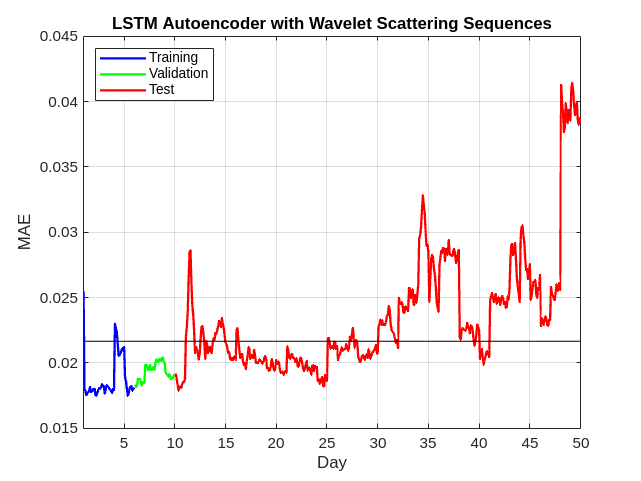There is an initial indication of anomalous behavior between day 11 and 12. Subsequently, the wind turbine appears to display anomalous behavior almost continually after day 30. This is consistent with the cumulative plot of the data.

One advantage of the LSTM autoencoder with wavelet scattering features is the significant data reduction wavelet scattering provides in the time dimension, from 48,828 samples down to 48. This enables us to train the autoencoder in less than 2 minutes using a GPU. Because training robust deep learning models often involves tuning hyperparameters, having a model which trains quickly is a significant advantage.

Conversely, training an autoencoder on the raw data required over 1.5 hours using a GPU. Accordingly, the training of the autoencoder with raw data is not repeated in this example. Here only the results are presented. The following LSTM autoencoder was trained on the raw data.

```Nt = length(rawfeatures{1}); lstmAutoEncoder = [ sequenceInputLayer(1, Normalization = "zscore", ... Name = "input", MinLength = Nt) lstmLayer(32, Name = "lstm1a") reluLayer(Name = "relu1") lstmLayer(16, Name = "lstm2a", OutputMode = "last") dropoutLayer(0.2, Name = "drop1") reluLayer(Name = "relu2") repeatVectorLayer(Nt) lstmLayer(16, Name = "lstm2b") dropoutLayer(0.2,Name = "drop2") reluLayer(Name = "relu3") lstmLayer(32, Name = "lstm1b") reluLayer(Name = "relu4") fullyConnectedLayer(1) regressionLayer(Name = "regression") ]; ```

Due to computational considerations, the number of hidden units in the LSTM layers was reduced with the raw data. Otherwise, the networks used with raw data and with wavelet scattering sequences were identical.

The threshold was determined in the exact same way as previously described. The following figure shows the results.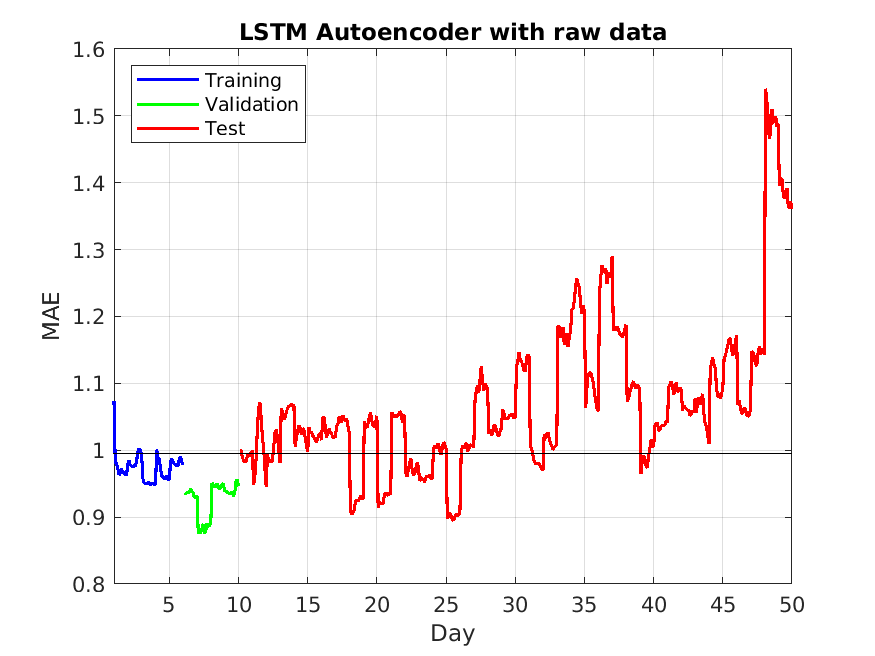There are several similarities between the results obtained with the wavelet scattering sequences and the raw data. Both show the data obtained between day 11 and 12 as an outlier. Both also indicate anomalous behavior with increasing regularity after approximately day 30. However, the autoencoder on the raw data appears to have underfit the training data and a few of the training records appear as anomalous. This does not agree with our knowledge that the wind turbine was functioning normally at the start of the recording period. There are also many more anomalies indicated by using the autoencoder on the raw data. Given the false positives on the training data, there is cause to suspect there are a number of false positives among these detections. Given that we have used a conservative estimate, it is likely that other thresholding methods would yield even more detections.

### Convolutional Autoencoder

While recurrent networks (RNNS) are powerful architectures for anomaly detection, RNNs are computationally expensive when the time dimension of the data becomes large. To reiterate, the LSTM autoencoder used above was computationally efficient because of the use of the wavelet scattering transform which reduced the time dimension of the data from 48,828 samples to 48 samples. As a result, training the autoencoder required less than two minutes using a GPU. On the other hand, training an LSTM on the raw data required more than 1.5 hours. The training discrepancies between the two approaches can be lessened by using a convolutional network. The convolutional network mimics the LSTM autoencoder by using convolutional layers with downsampling at the encoding stage followed by transposed convolutional layers with upsampling at the decoding stage.

Create a convolutional network. Normalize the data at the input layer using Z-score normalization. To downsample the input, specify repeating blocks of 1-D convolution, ReLU, and dropout layers. To upsample the encoded input, include the same number of blocks of 1-D transposed convolution, ReLU, and dropout layers.

For the convolution layers, specify decreasing numbers of filters with size 11. To ensure that the outputs are downsampled evenly by a factor of 2, specify a stride of `2`, and set the `Padding` option to `"same"`. For the transposed convolution layers, specify increasing numbers of filters with size 11. To ensure that the outputs are upsampled evenly be a factor of 2, specify a stride of `2`, and set the `Cropping` option to `"same"`.

For the dropout layers, specify a dropout probability of 0.2. To output sequences with the same number of channels as the input, specify a 1-D transposed convolution layer with the number of filters matching the number of channels of the input. To ensure output sequences are the same length as the layer input, set the `Cropping` option to `"same"`. At the output use a regression layer.

```numDownsamples = 2; minLength = 48; filterSize = 11; numFilters = 16; dropoutProb = 0.2; numChannels = npaths; convlayers = [ sequenceInputLayer(numChannels,Normalization="zscore",... MinLength=minLength,Name = 'InputLayer') % Encoder stage convolution1dLayer(filterSize,2*numFilters,Padding="same",Stride=2) reluLayer dropoutLayer(dropoutProb,Name = "dropout1") convolution1dLayer(filterSize,numFilters,Padding="same",Stride=2) reluLayer dropoutLayer(dropoutProb,Name = "dropout2") % Decoder stage transposedConv1dLayer(filterSize,numFilters,Cropping="same",Stride=2) reluLayer dropoutLayer(dropoutProb,Name = "transpdropout1") transposedConv1dLayer(filterSize,2*numFilters,Cropping="same",Stride=2) reluLayer dropoutLayer(dropoutProb,Name = "transpdropout2") % Make channels agree for output transposedConv1dLayer(filterSize,numChannels,Cropping="same", ... Name = "FinalConvLayer") regressionLayer('Name','regression') ];```

Train the convolutional network for 300 epochs. Shuffle the training data on each epoch. Output the network with the best validation loss.

```options = trainingOptions("adam", ... MaxEpochs=300, ... Shuffle="every-epoch", ... ValidationData={validationscatFeatures,validationscatFeatures}, ... Verbose=0, ... OutputNetwork = 'best-validation-loss',... Plots="training-progress"); convNetSCAT = trainNetwork(trainscatFeatures,trainscatFeatures,convlayers,options);```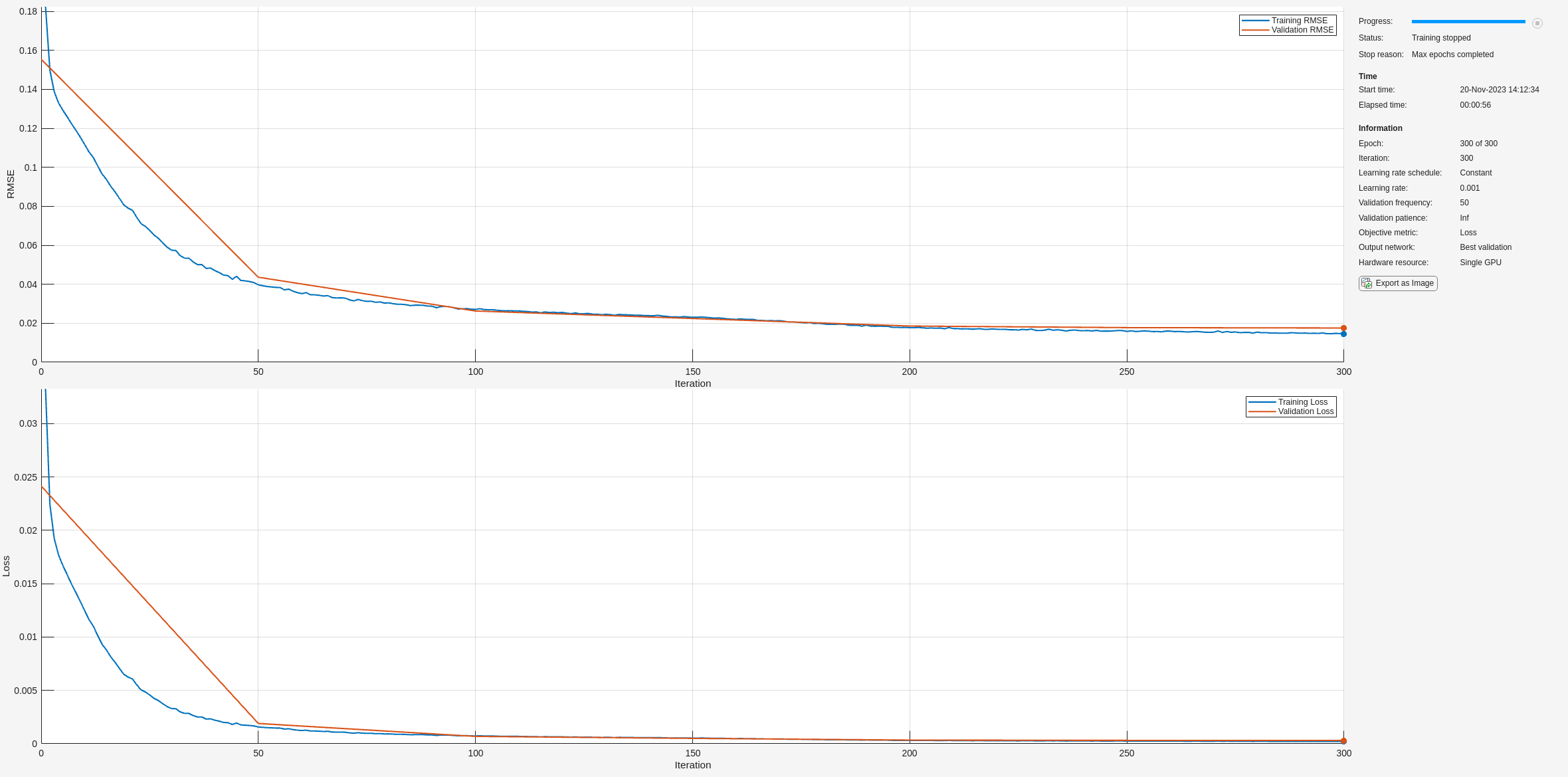Calculate the MAE losses as done with the LSTM autoencoder.

```ypredCTrain = cellfun(@(x)predict(convNetSCAT,x),trainscatFeatures,'UniformOutput',false); maeCTrain = cellfun(@(x,y)maeLoss(x,y),ypredCTrain,trainscatFeatures); ypredCValidation = cellfun(@(x)predict(convNetSCAT,x),validationscatFeatures,'UniformOutput',false); maeCValid = cellfun(@(x,y)maeLoss(x,y),ypredCValidation,validationscatFeatures); ypredCTest = cellfun(@(x)predict(convNetSCAT,x),testscatFeatures,'UniformOutput',false); maeCTest = cellfun(@(x,y)maeLoss(x,y),ypredCTest,testscatFeatures); if useGPU [maeCTrain,maeCValid,maeCTest] = gather(maeCTrain,maeCValid,maeCTest); end```

Use only the validation data to determine the threshold for anomaly detection. Utilize the same threshold determination method as used with the LSTM autoencoder.

`threshCV = quantile(maeCValid,0.75)+1.5*iqr(maeCValid);`

Plot the results.

```figure plot(... (1:length(maeCTrain))/11,maeCTrain,'b',... (length(maeCTrain)+[1:length(maeCValid)])/11,maeValid,'g',... (length(maeCTrain)+length(maeCValid)+[1:length(maeCTest)])/11,maeTest,'r',... 'linewidth',1.5) hold on plot((1:550)/11,thresh*ones(550,1),'k') hold off xlabel("Day") ylabel("MAE") xlim([1 50]) legend("Training","Validation","Test","Location","NorthWest"); title("Convolutional Network with Wavelet Scattering Sequences") grid on```Use the same convolutional network to work on the raw data. Change the number of channels to 1 to match the raw data dimensions. Training with a dropout probability of 0.2 resulted in an severe underfitting of the training data. As a result, reduce the dropout probability to 0.1. Otherwise, the networks used with the wavelet scattering sequences and the raw data are identical.

```lgraph = layerGraph(convlayers); inputlayer = sequenceInputLayer(1,Normalization="zscore",... MinLength=48,Name = 'InputLayerRaw'); doLayer = dropoutLayer(0.1); tconvLayer = transposedConv1dLayer(filterSize,1,Cropping="same", ... Name = "FinalConvLayer"); rawConvLayers = replaceLayer(lgraph,"InputLayer",inputlayer); rawConvLayers = replaceLayer(rawConvLayers,"dropout1",doLayer); rawConvLayers = replaceLayer(rawConvLayers,"dropout2",doLayer); rawConvLayers = replaceLayer(rawConvLayers,"transpdropout1",doLayer); rawConvLayers = replaceLayer(rawConvLayers,"transpdropout2",doLayer); rawConvLayers = replaceLayer(rawConvLayers,"FinalConvLayer",tconvLayer);```

Train the network on the raw data. Use the same options are used with the wavelet scattering sequences.

```options = trainingOptions("adam", ... MaxEpochs=300, ... Shuffle="every-epoch", ... ValidationData={validationrFeatures,validationrFeatures}, ... Verbose=0, ... OutputNetwork = "best-validation-loss",... Plots="training-progress"); convNetRAW = trainNetwork(trainrFeatures,trainrFeatures,rawConvLayers,options);```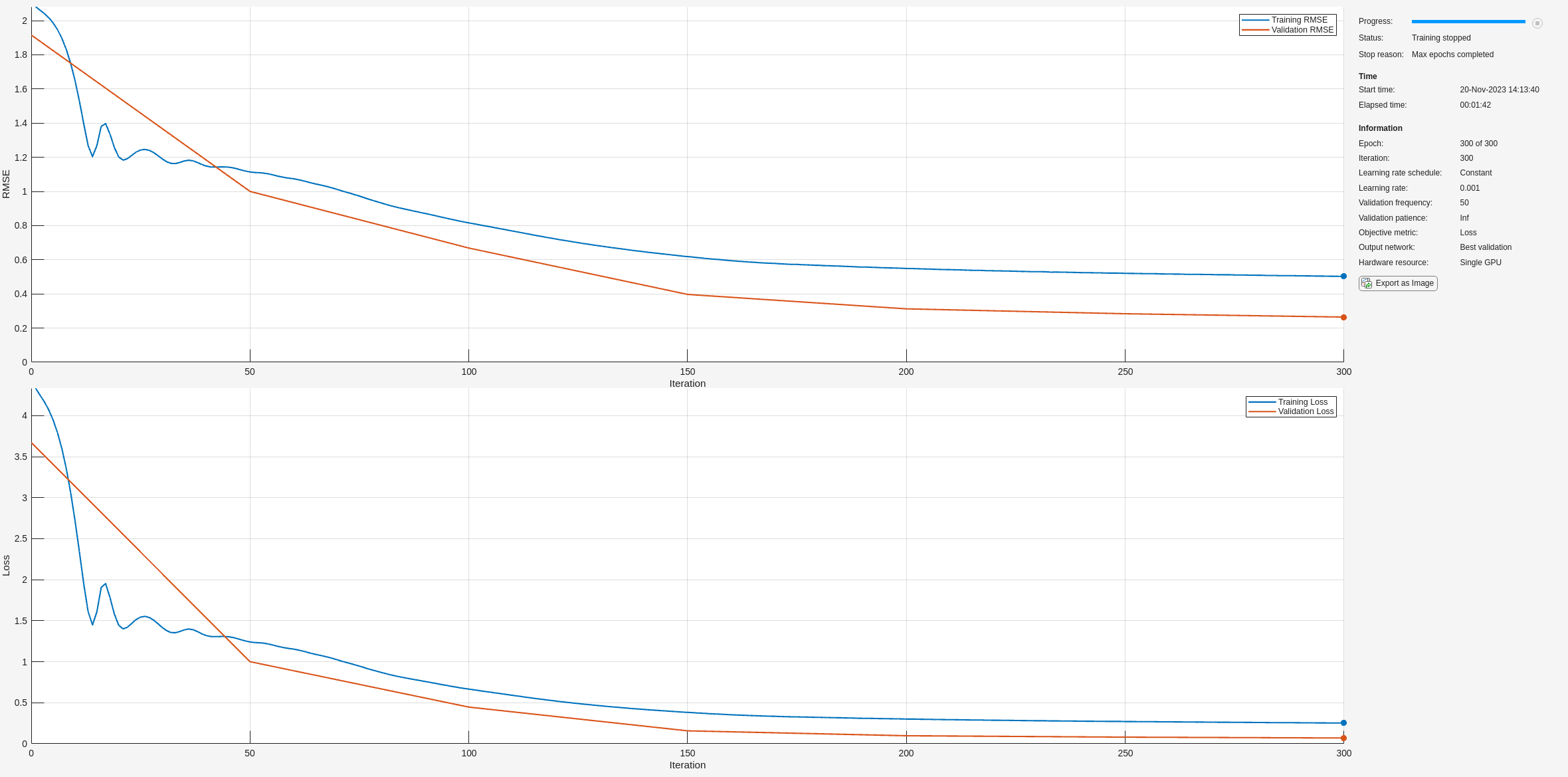Calculate the MAE losses and determine the threshold.

```ypredRTrain = cellfun(@(x)predict(convNetRAW,x),trainrFeatures,'UniformOutput',false); maeRTrain = cellfun(@(x,y)maeLoss(x,y),ypredRTrain,trainrFeatures); ypredRValidation = cellfun(@(x)predict(convNetRAW,x),validationrFeatures,'UniformOutput',false); maeRValid = cellfun(@(x,y)maeLoss(x,y),ypredRValidation,validationrFeatures); ypredRTest = cellfun(@(x)predict(convNetRAW,x),testrFeatures,'UniformOutput',false); maeRTest = cellfun(@(x,y)maeLoss(x,y),ypredRTest,testrFeatures); if useGPU [maeRTrain,maeRValid,maeRTest] = gather(maeRTrain,maeRValid,maeRTest); end threshCVraw = quantile(maeRValid,0.75)+1.5*iqr(maeRValid);```

Plot the results.

```figure plot(... (1:length(maeRTrain))/11,maeRTrain,'b',... (length(maeRTrain)+[1:length(maeRValid)])/11,maeRValid,'g',... (length(maeRTrain)+length(maeRValid)+[1:length(maeRTest)])/11,maeRTest,'r',... 'linewidth',1.5) hold on plot((1:550)/11,threshCVraw*ones(550,1),'k') hold off xlabel("Day") ylabel("MAE") xlim([1 50]) legend("Training","Validation","Test","Location","NorthWest"); title("Convolutional Network with Raw Data") grid on```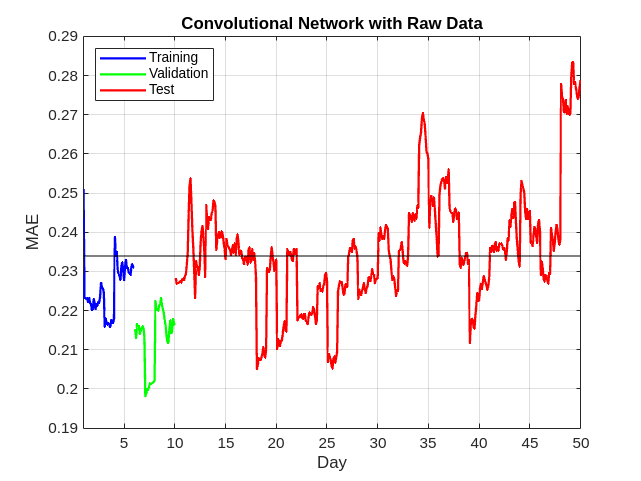Note that using the convolutional autoencoder has reduced the training time for the wavelet scattering sequences from a little under one and a half minutes to approximately 45 seconds using a GPU. The most significant training time input has occurred for the raw data, where training the LSTM autoencoder required approximately 1.5 hours while the convolutional network completed the training in approximately 3 minutes.

With respect to the results, those obtained with the convolutional network are similar to the LSTM autoencoder for both the raw data and wavelet scattering sequences. In agreement with the LSTM autoencoder results, the wavelet scattering based convolutional autoencoder exhibits anomalous behavior between day 11 and 12. The wind turbine's behavior returns to the normal range again until about day 30 when detections of anomalous behavior begin to occur with increasing frequency.

The results for the raw data are also similar to those obtained with the LSTM autoencoder. There are detections in the training data, which are likely indicative of false detections. This causes some suspicion of the detections which occur for the raw data networks during the early portion of the recording near day 15.

It is important to note that preliminary analysis of records indicated as anomalous by both methods did not reveal any clear differences using conventional signal analysis techniques such as the short-time Fourier transform or continuous wavelet transform.

### Discussion

In this example, we used both wavelet scattering sequences and raw data with two types of deep autoencoders to detect inner-race faults in a wind turbine. The use of the wavelet scattering sequences in place of raw data offered some advantages. First, it greatly reduced the dimensionality of the problem along the time dimension. This allows for more rapid prototyping of models including optimization of hyperparameters. Because this is such a critical part of the successful application of deep learning, it is hard to overstate this advantage with respect to LSTM autoencoders. Secondly, the deep networks trained on wavelet scattering sequences seem to be more robust against false detections.

The convolutional autoencoder provided a training-time advantage for both the wavelet scattering sequences and the raw data, but the relative advantage was far more significant with the raw data. Using the convolutional autoencoder with both sets of features provides ample opportunity for optimization of hyperparameters in a reasonable amount of time.

Finally, the use of these different networks and different features are also potentially complementary. Specifically, they offer the possibility to more closely investigate those records which all methods designate as normal vs. anomalous with more detailed signal analysis techniques. In an unsupervised learning problem, this allows us to increase our understanding of the data and develop even more powerful models for machine monitoring.

### References

 Bechhoefer, Eric, Brandon Van Hecke, and David He. 2013. “Processing for Improved Spectral Analysis”. Annual Conference of the Prognostics and Health Management Society 5 (1).

### Supporting Functions

```function mae = maeLoss(ypred,target) mae = mean(abs(ypred-target),'all'); end```